Contact

1. TOP
2. Products
3. SC/Tetra

SC/Tetra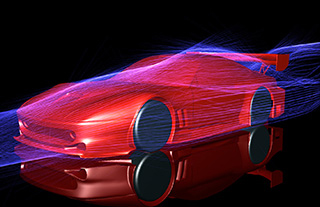Features

SC/Tetra is general purpose thermo-fluid simulation software using hybrid mesh to represent the surface shape with high accuracy. Its characteristics include sophisticated mesh generation system, high speed computing, low memory consumption, and user-friendly features throughout the operation. You can obtain simulation results in a short time without stress.

Program Structure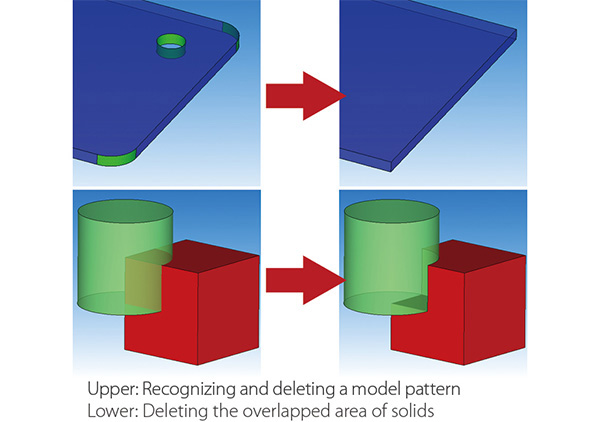When CAD data to be used for simulation has a problem, the data can be modified with Preprocessor. Boundary conditions can be set based on the part names and color information set in the CAD data. When some regions are missing in the model, shapes such as cuboids and cylinders can be added.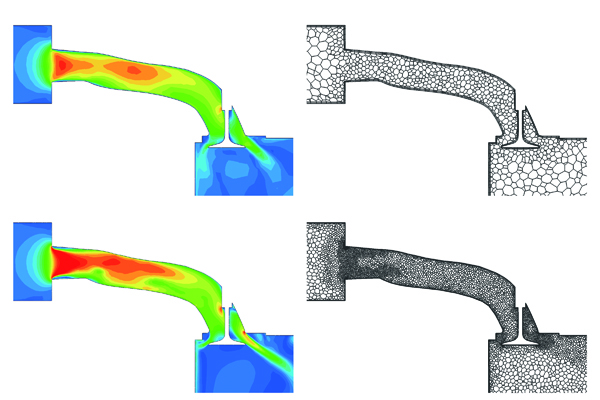With this function, mesh will be automatically refined where a flow or pressure changes greatly in a steady-state analysis. After the calculation in Solver is completed, Preprocessor automatically launches and executes gridding and meshing based on the calculation result. By specifying the target number of elements, coarse mesh is generated first and the mesh is automatically refined to be appropriate for the calculation. The function is useful for an analysis of flows in a tube with a complicated shape.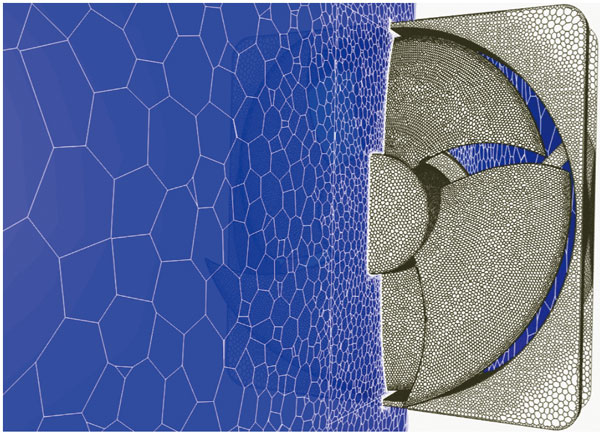Discontinuous mesh

Flow with object motion can be calculated, including rotation of fans and turbines, and crossing travel of automobiles or trains (translation). The function enables an analysis with consideration on shear heating between rotor and pad in a disk brake. The function also makes it possible to analyze a combination of rotation and translation such as a piston pump.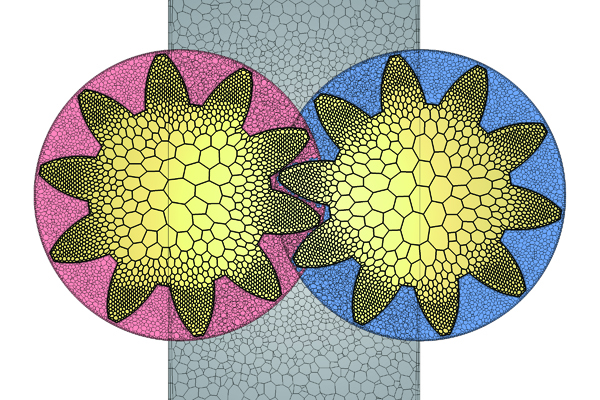Overset mesh

Free movement of regions, that cannot be analyzed using existing functions such as stretching or rotating elements, can now be simulated by overlapping mesh elements for stationary and moving regions. This function supports an overlap of multiple moving regions, a contact between objects, and a 6-degree-of-freedom motion of rigid bodies. This is useful to analyze opening and closing of a valve of an engine port or a gear pump where gears engage with each other.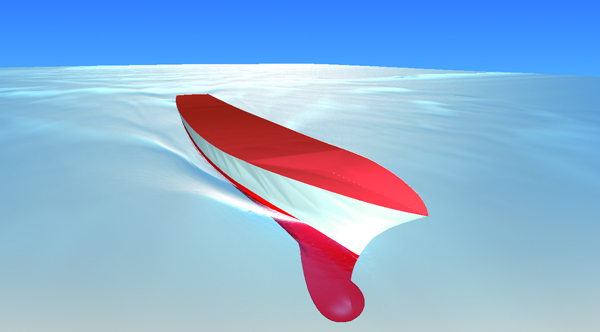Free surface (steady-state / transient)

The shape of an interface between a gas and a liquid can be simulated. Calculations by VOF method (new method: FIRM) are fast and accurate, and functions including moving boundary, overset mesh, and particle tracking can be used in combination. Because a phenomenon where the phase interface becomes stable can be analyzed in a steady-state calculation, the result can be obtained in a shorter time than before.

* Only scFLOW supports FIRM.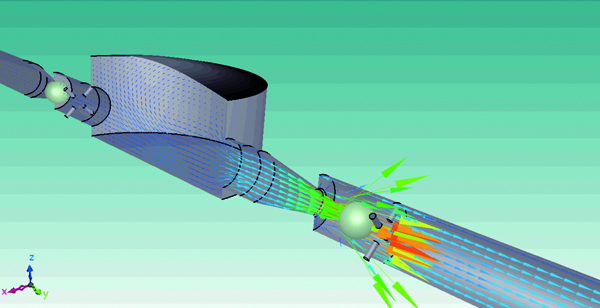6-degree-of-freedom motion (6DOF)

Passive translation and rotation of a rigid body receiving a fluid force can be analyzed. With the function, the user can analyze a ball valve with consideration of the elasticity of the spring (1D translation), and paper airplane with consideration of 6-degree-of-freedom rigid-body motion (3D translation + 3D rotation). In addition, the function is applied to analyses of check valves, wind power generators, and blades of wave power generators.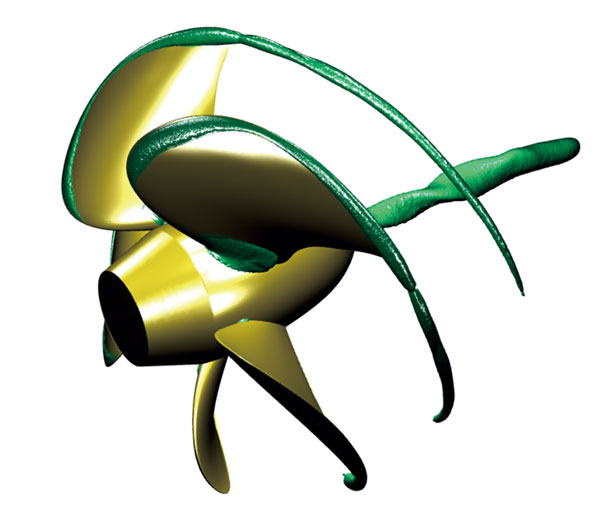Cavitation

This function enables simulation of a vaporization phenomenon called cavitation, which is caused at an area where pressure of a liquid becomes lower than in the surrounding area, such as with a propeller rotating at a high speed under water. The occurrence of cavitation can be predicted by applying the cavitation model based on the pressure values. The software also supports problems caused by cavitation such as erosion.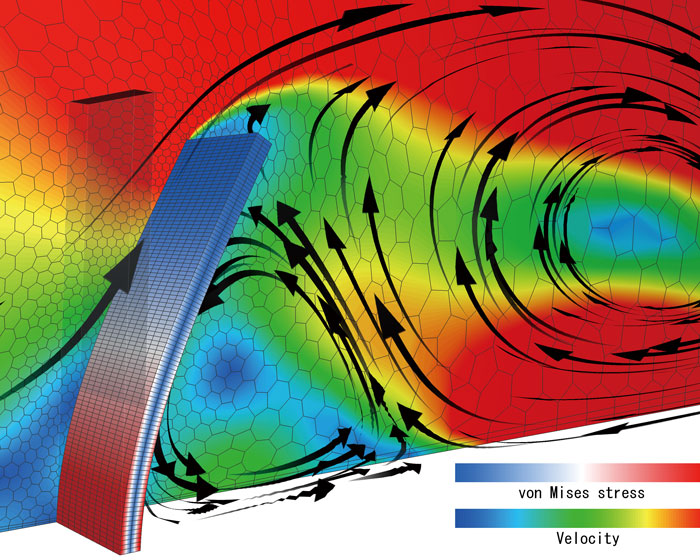Fluid-structure interaction

This option is used for two-way FSI (fluid-structure interaction) with structural analysis software. With this option, not only rigid bodies but also elastic bodies can be treated. Deformation of an object caused by a fluid force and the change of fluid caused by the deformation can be analyzed.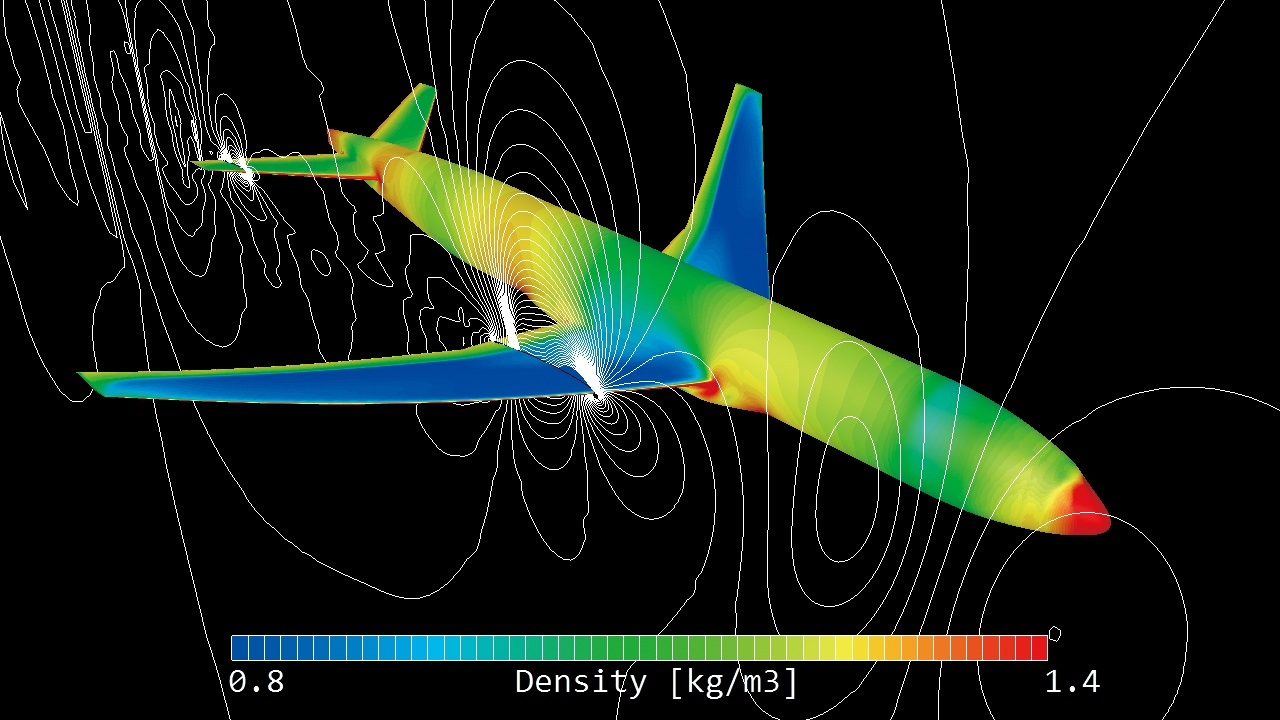Compressible fluid

The software can analyze phenomena such as supersonic flow and significant expansion/contraction of volume. For a compressible fluid, both the pressure-based and the density-based Solvers can be used. The density-based Solver keeps the calculation stable even with high Mach number. You can select either Solver depending on the analysis target and phenomenon.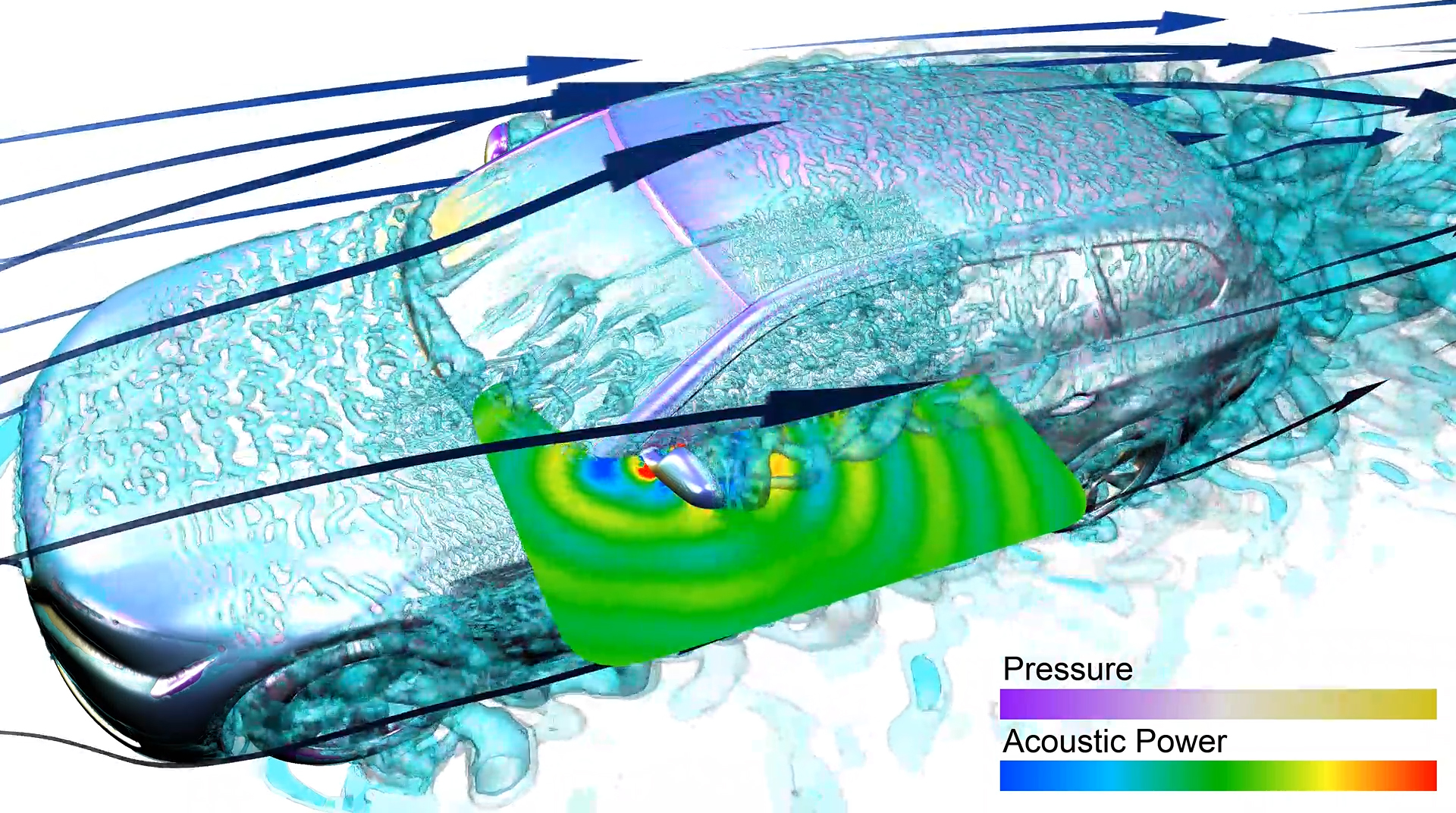Aerodynamic noise analysis

Sound caused by pressure oscillation of a fluid, such as wind noise, and sound caused by resonance can be predicted. The calculation can be performed accurately by using LES and the weak compressible flow model. The frequency of aerodynamic noise can also be analyzed using the Fast Fourier Transform (FFT) method from the CFD analysis result.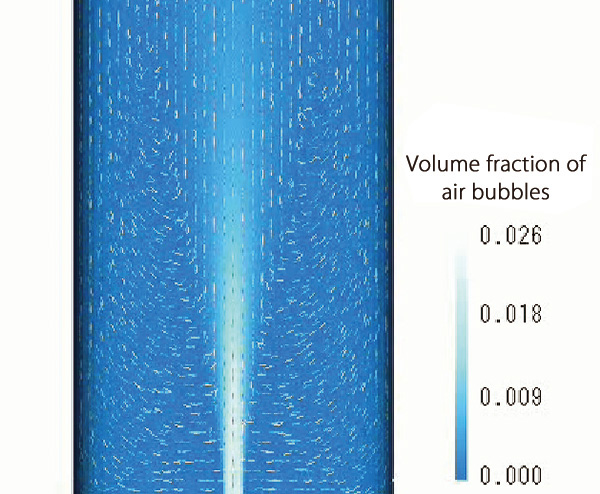Dispersed multi-phase flow

This function can simulate flows containing many bubbles, droplets, or particles (dispersed phase), which are difficult to be analyzed using free surface. This function is a multi-fluid model that can predict volume fraction distribution and velocity distribution of each phase by solving the governing equation under the assumption that the dispersed phase is a fluid (continuous phase). The function is useful to analyze the bubble jet effect and aeration tanks.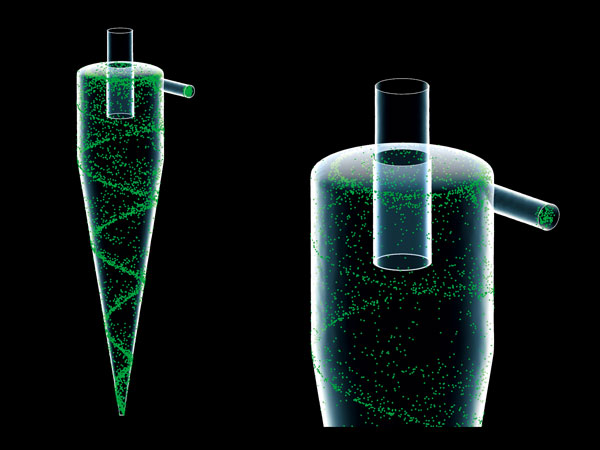Particle tracking

Particle tracking function enables analyzing behavior of particles in flow. When analyzing small particles that follow the fluids movement (such as steam and dust), marker particle function can be used to evaluate particles in flow that change over time, which assumes that particle movement is in accordance with fluid velocity.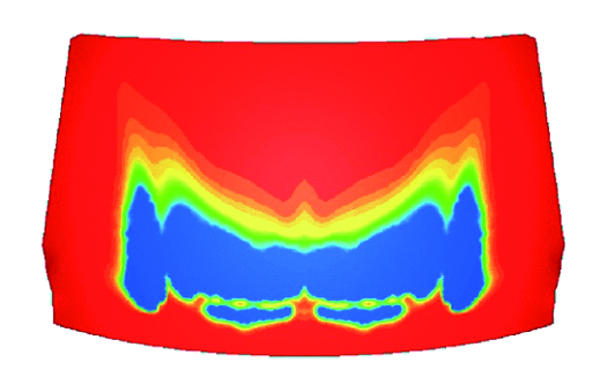Humidity dew condensation

The amount of dew condensation on an object surface can be calculated from the surface temperature and water vapor in the air. You can output the amount of dew condensation per unit time in a steady-state analysis and the accumulated dew condensation in a transient analysis. Evaporation from a surface where dew condensation occurs can be calculated simultaneously, and this is useful for an analysis of a windshield defroster.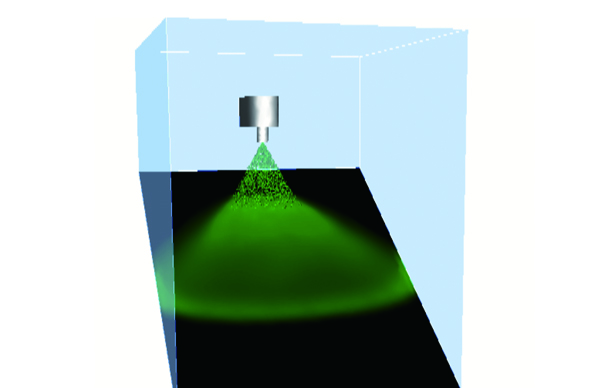Liquid film model

The liquid film model is an extended function of the particle tracking function. By using the model, you the user can simulate the phenomenon that liquid particles change to a liquid film (water on a wall) when they reaching on the a wall. A liquid film on a wall flows with the influence of gravity and a gas-phase flowdown depending on an angle of the wall and collects in at a certain position. The analysis results are output as the thickness of a liquid film.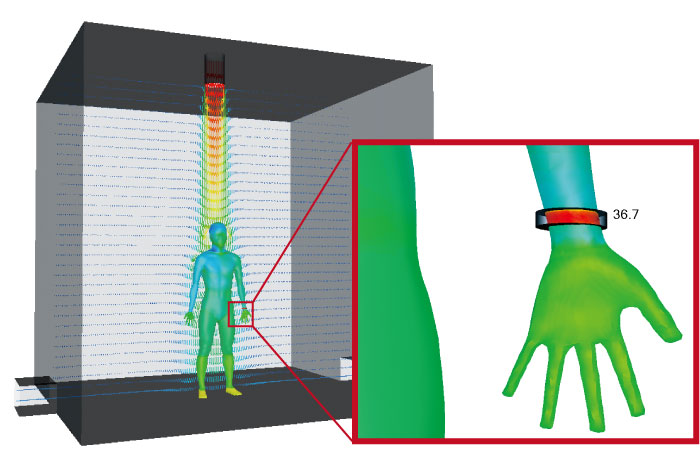Thermo-regulation model (JOS)

Combination use of the thermoregulation-model (JOS) and a fluid analysis enables analyses of the surface temperature of a human body under a certain thermal environment. It can also be used to analyze temperature and humidity changes in the surrounding environment of a human body. The user can consider age, clothes, and physiological phenomena of the human body such as heat transfer by blood flow in addition to the surrounding environment of a human body such as temperature and velocity.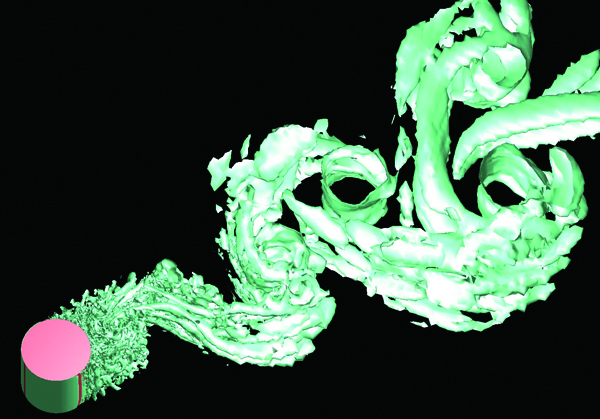LES

LES is one of the turbulent flow models. It models eddies smaller than the mesh element in size and directly calculates other eddies. Although calculation load is large, LES enables simulations closer to real phenomena. LES is often used in noise analyses, significantly affected by time variation, to simulate the behavior of small eddies. The user can use the hybrid model with RANS, a turbulent model of small calculation load.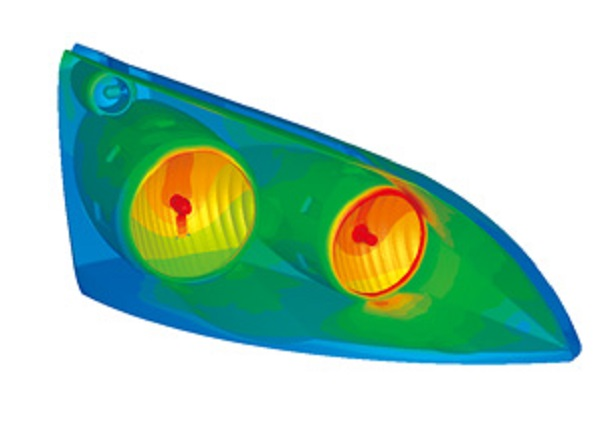Heat transfer by infrared-ray radiation can be considered by setting emissivity and temperature difference between objects. The user can choose VF (view factor) method or FLUX method as a calculation method. The user can also consider wavelength dependence, transmission, absorption, refraction, diffusion, and reflection of radiation. In FLUX method, the user can also consider directionality.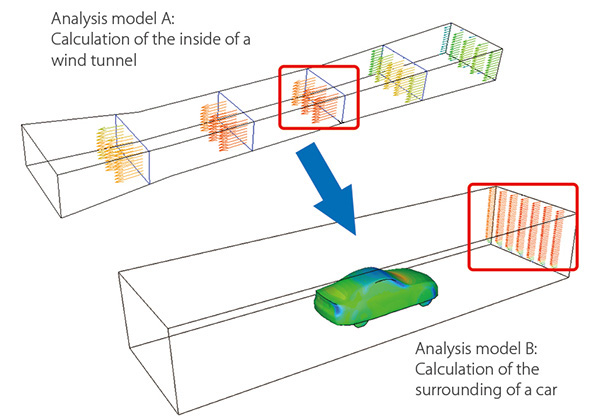Mapping

When a target phenomenon is in a small range and the phenomenon is affected by a wide range of its surrounding area, analysis results of the surrounding area can be used for an analysis of the target phenomenon as boundary conditions to decrease the calculation load.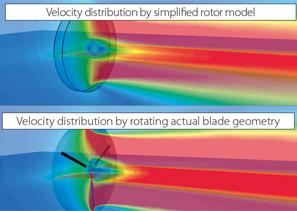Fan model (rotating blades)

With this model, an average flow field around rotating blades can be simulated only by entering characteristic properties regardless of real shapes of fans or propellers. The user can use the non-dimensional swirl coefficient model, the simplified propeller model, and the simplified rotor model. This model is useful to analyze axial-flow windmills and waterwheels.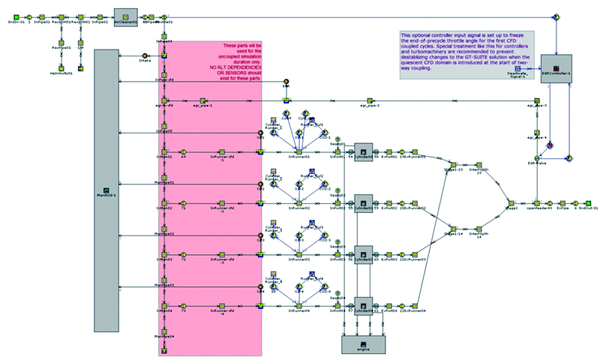Coupled analysis with GT-SUITE

The amount of dew condensation on an object surface can be calculated from the surface temperature and water vapor in the air. You can output the amount of dew condensation per unit time in a steady-state analysis and the accumulated dew condensation in a transient analysis. Evaporation from a surface where dew condensation occurs can be calculated simultaneously, and this is useful for an analysis of a windshield defroster.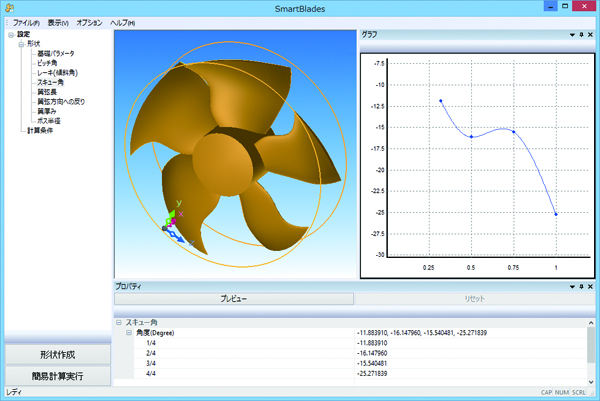This function is useful for analyzing the shape of a fan automatically throughout creating the shape of a fan (CAD data), calculating the flow, and post-processing. The shape of a fan can be created easily by specifying parameters including the number of blades, fan diameter, rake angle, and skew angle.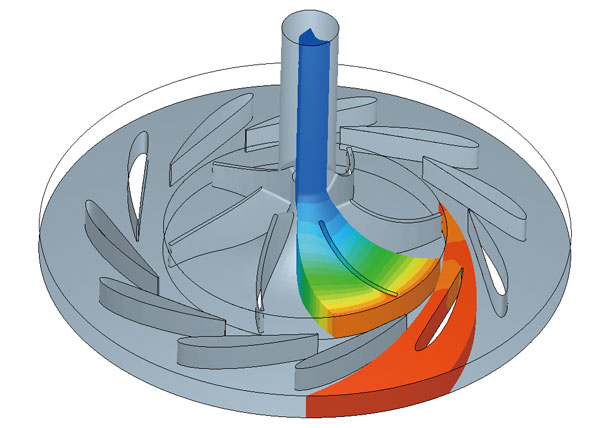Functions for turbomachinery

One-pitch shape can be extracted from a periodic model such as an impeller or a vane of turbomachinery. The analysis result of the one-pitch model can be checked in the meridian plane. Two regions whose pitches are different can also be analyzed. The calculation load will be reduced by using this function.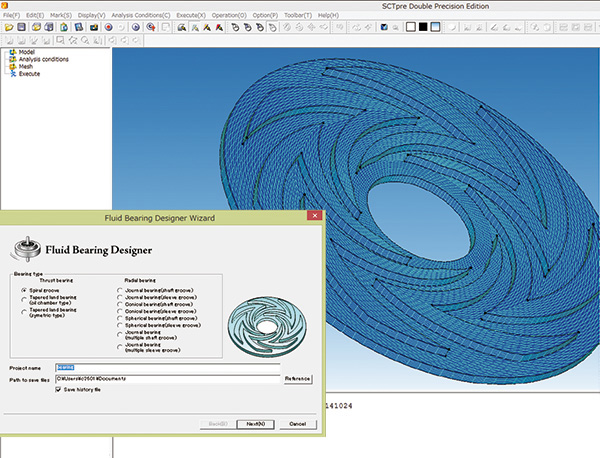FluidBearingDesigner

The function creates groove patterns of fluid bearings (dynamic-pressure bearing) and generates mesh. You can select the shape of grooves such as journal and thrust and materials such as porous material. From calculation results, you can obtain parameters for designing fluid bearings such as axial force and drag coefficient.